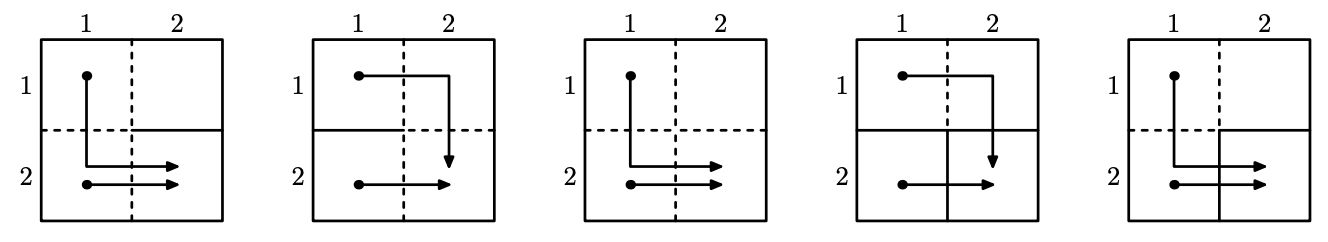시간 제한 메모리 제한 제출 정답 맞은 사람 정답 비율
1 초 512 MB 0 0 0 0.000%

## 문제

The annual race of robots takes place today in Berland. Ivan is one of it's participants. Before the race starts, he decided to find out how does the racing field look like.

Racing field is a rectangle of size $n \times m$ divided into $nm$ unit cells. Rows of the rectangle are numbered from $1$ to $n$, columns are numbered from $1$ to $m$. We denote as $(i, j)$ the cell located at the row $i$ and the column $j$. There can be a barrier between each pair of adjacent cells.

There are $n$ robots participating in the race. Before the race starts, they are located in cells of the first column: $(1, 1), (2, 1), \ldots, (n, 1)$. Finish is located in the cell $(n, m)$. When the race begins, each robot starts moving towards the finish. From the cell $(i, j)$ robot can move either to the cell $(i + 1, j)$ or to the cell $(i, j + 1)$. If the robot is moving between cells not separated by a barrier, it moves between these cells immediately. Otherwise, it takes one second to move between them.

Each robot knows locations of all barriers in advance and is moving to the finish cell using the path that needs minimum time to follow. Note that robots are very small, so any number of them may stay in one cell during the race. We denote the field fair, if the time needed to reach the finish is equal for all robots.

Ivan managed to take a look on the racing field and remembered some information about it. Namely, for some pairs of adjacent cells he definitely knows whether there is a barrier between them or not. Also he is sure that the racing field is fair. Ivan is wondering to know, how many fields satisfying all these requirements do exist. Unfortunately, now he is busy preparing to the competition, so he asked you to figure it out. As the answer might be very huge, Ivan only wants to know it modulo $998244353$.

## 입력

The first line of input contains two integers $n$ and $m$ --- the size of the field ($1 \le n \le 5$, $1 \le m \le 15$).

The second line contains a single integer $k$ --- the number of pairs of cells Ivan knows the information about ($0 \le k \le 2nm - n - m$).

Each of the next $k$ lines contains five integers $r_1$, $c_1$, $r_2$, $c_2$ and $w$ ($1 \le r_1 \le r_2 \le n$, $1 \le c_1 \le c_2 \le m$, $0 \le w \le 1$). If $w = 0$, then there is no barrier between cells $(r_1, c_1)$ and $(r_2, c_2)$. If $w = 1$, then there is a barrier between these cells. It's guaranteed, that cells $(r_1, c_1)$ and $(r_2, c_2)$ are adjacent. It's guaranteed, that no pair of cells will be mentioned in the input more than once.

## 출력

Output single integer --- the number of fair fields, satisfying all Ivan's conditions, modulo $998244353$.

## 예제 입력 1

2 2
1
1 1 1 2 0


## 예제 출력 1

5


## 힌트

Illustration for the sample test. Solid lines denote a barrier, dashed lines denote its absence.